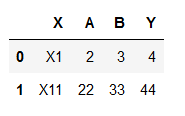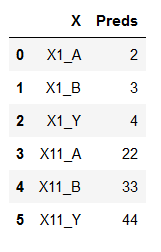Converting Pandas dataframe

(Akash ) #1

I have a dataframe as belowI would like to convert it into the following dataframehow can i do this?

0 Likes

(Akash ) #2

Edit 1: I figured out how to do this. Pandas has a stack function which can do this function. Also check out pivot.

0 Likes

(Kevin Bird) #3

Would you be able to post the code solution you used to fix this for future people that may have this same type of situation?

0 Likes

(Akash ) #4

SureTry this :

stacked = df.stack()
stacked = stacked.to_frame()
stacked = stacked.reset_index()
stacked= stacked.drop(['level_0'], axis = 1)
0 Likes